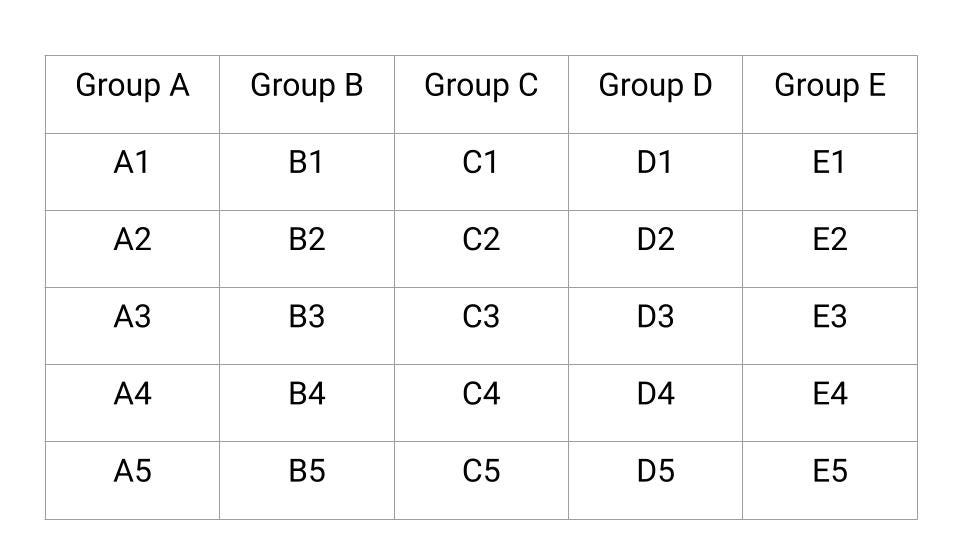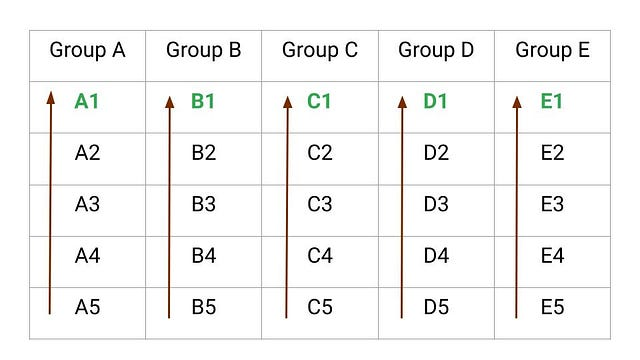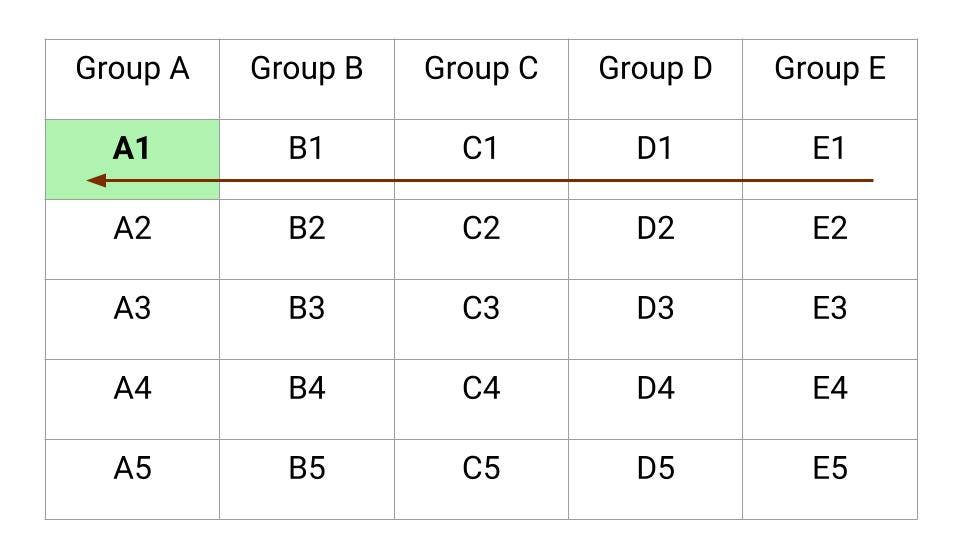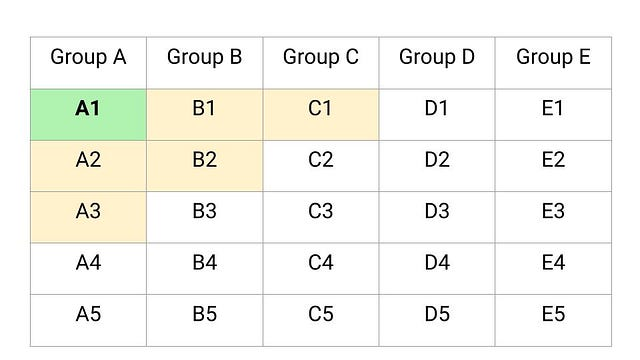# Find out the Fastest 3 Horses

### Problem Statement

There are 25 horses among which we need to find out the fastest 3 horses. In each race, only 5 horses can run simultaneously because there are only 5 tracks. What is the minimum number of races required to find the 3 fastest horses without using a stopwatch?

### Solution Idea and Steps

We can solve this problem using the idea of elimination. There are 25 horses, and we can only include 5 horses in a race. To know the relative speed of each horse, we need to include all the horses in atleast one race. Think! Here is the step-by-step strategy to identify the top 3 fastest horses.

Step 1: We divided the 25 horses into groups of 5 horses.Step 2: First 5 races

Now, we conduct a race in each group to know the relative speed of horses in each group. This would require 5 races. Let’s consider A1, B1, C1, D1, and E1 toppers of those 5 races.Step 3: 6th Race

Now we can easily decide the faster horse which would be the fastest among the winner of each group. So we make a group of 5 these horses (A1, B1, C1, D1, E1 ) and conduct a race among them. The winner of this race is the fastest horse overall. So after 6 races, we know the fastest horse. Suppose the result is A1 > B1 > C1 > D1 > E1. Now we move forward to find 2nd and 3rd fastest.Step 4: 7th Race

Now for the 2nd position: B1 and A2 will be the potential candidates. Similarly, for the 3rd position: A2, B1, C1, B2, and A3 are potential candidates. Overall, for the 2nd and 3rd positions: A2, B1, C1, B2, and A3 are the potential candidates.

• B1 and A2 may be second or third
• C1 or B2 OR A3 may be thirdIn the group of these 5 horses, the winner would be the 2nd fastest, and the horse that comes 2nd in the race would be the 3rd fastest!

So using this procedure, we need 7 races to decide the top 3 fastest horses. But the critical question is: why 7 races minimum? Let's think!

• To find the fastest, we need to run all 25 horses at least once, and since you can only race 5 horses at a time, you need a minimum of 25/5 = 5 races.
• Then we need to compare the winners of these races, which means a 6th race is necessary.
• To find the second and third fastest, we need at least one more race to compare the relative speed of the horses.

Another critical question is: can we generalize this strategy to n^2 horses and n tracks, where we want to find the fastest k horses (k < n)? Let's think!

Enjoy learning, Enjoy thinking!

Share on social media:

© 2020 Code Algorithms Pvt. Ltd.